CBSE Class 10 Sample Paper for 2023 Boards - Maths Standard

Class 10
Solutions of Sample Papers for Class 10 Boards

## (d) Assertion (A) is false but reason (R) is true.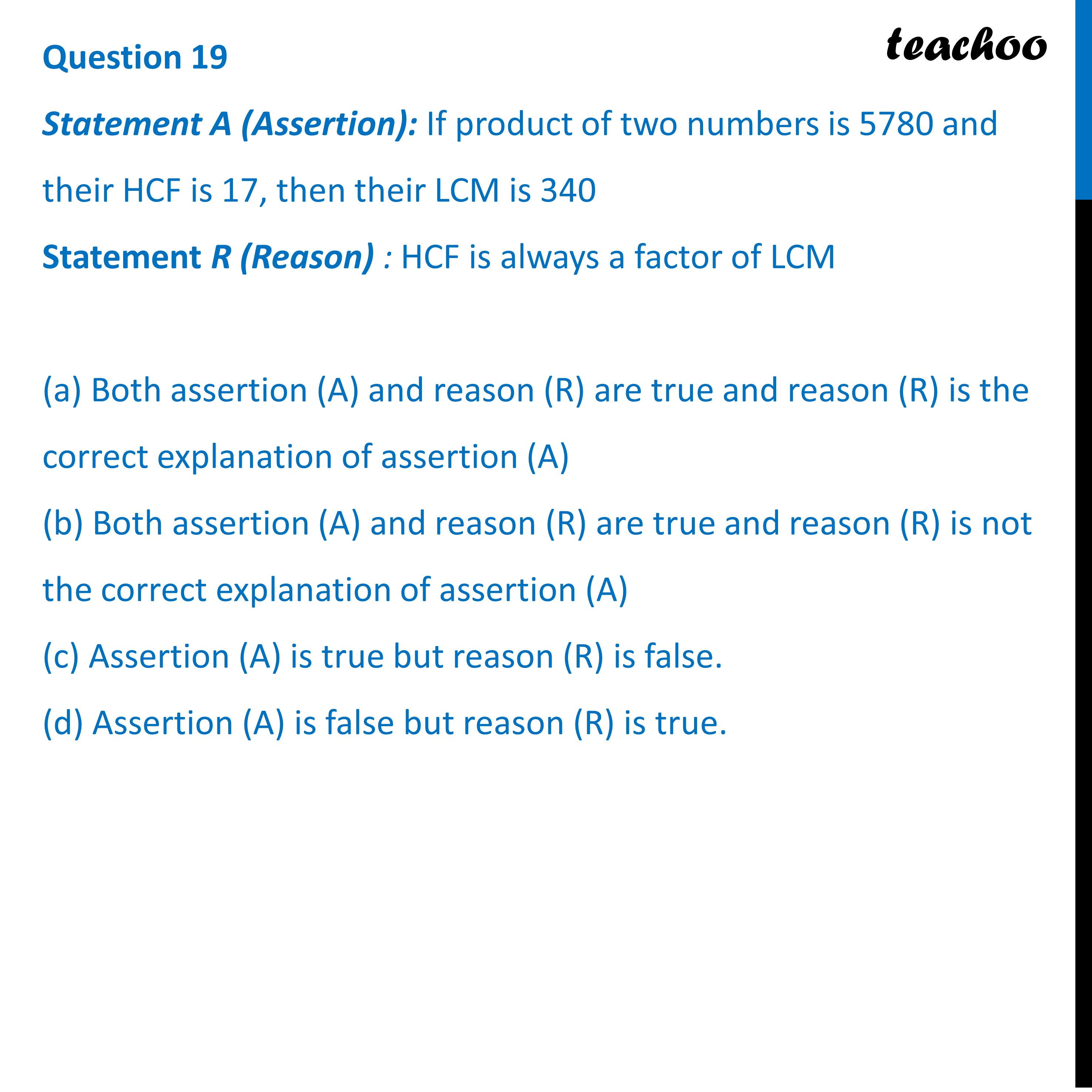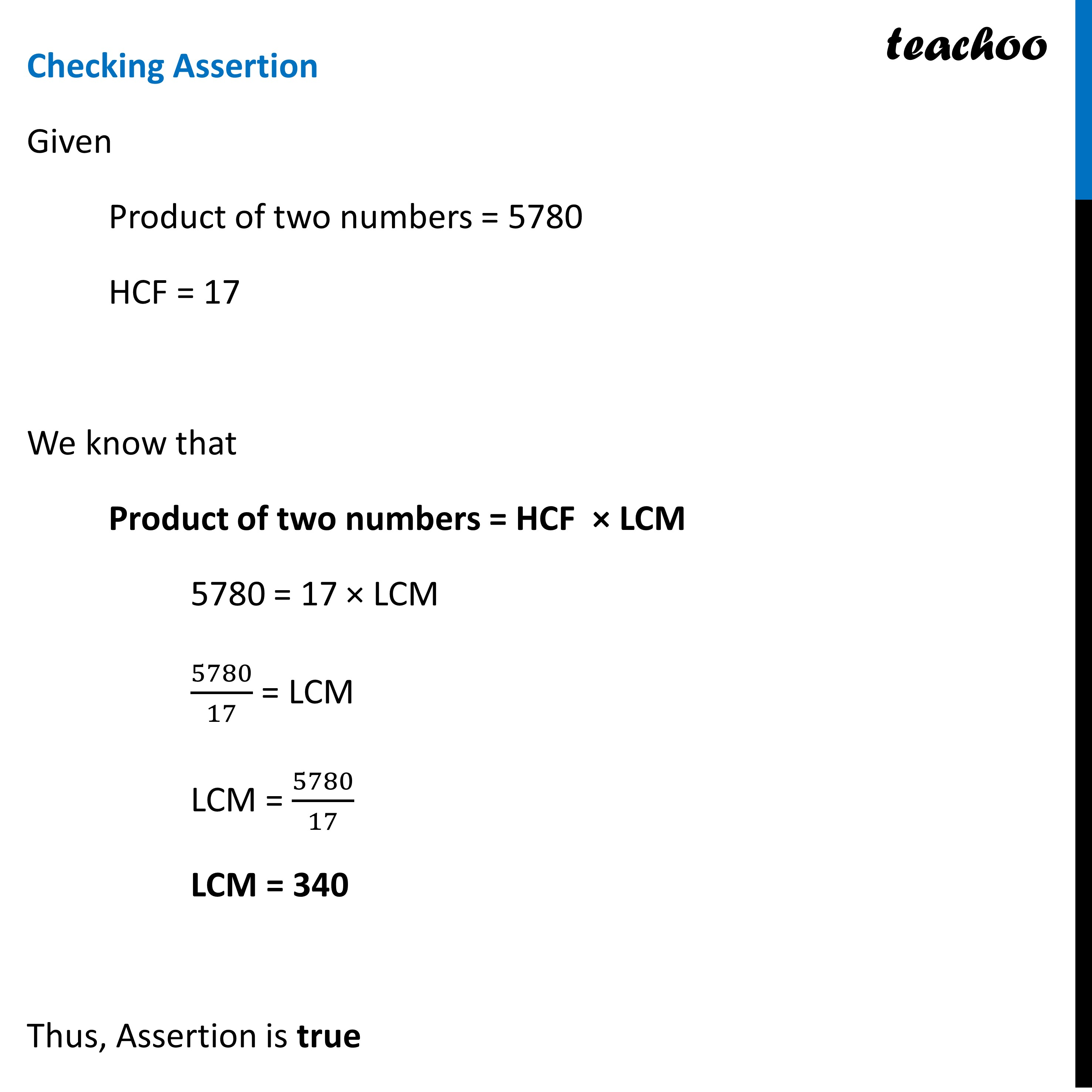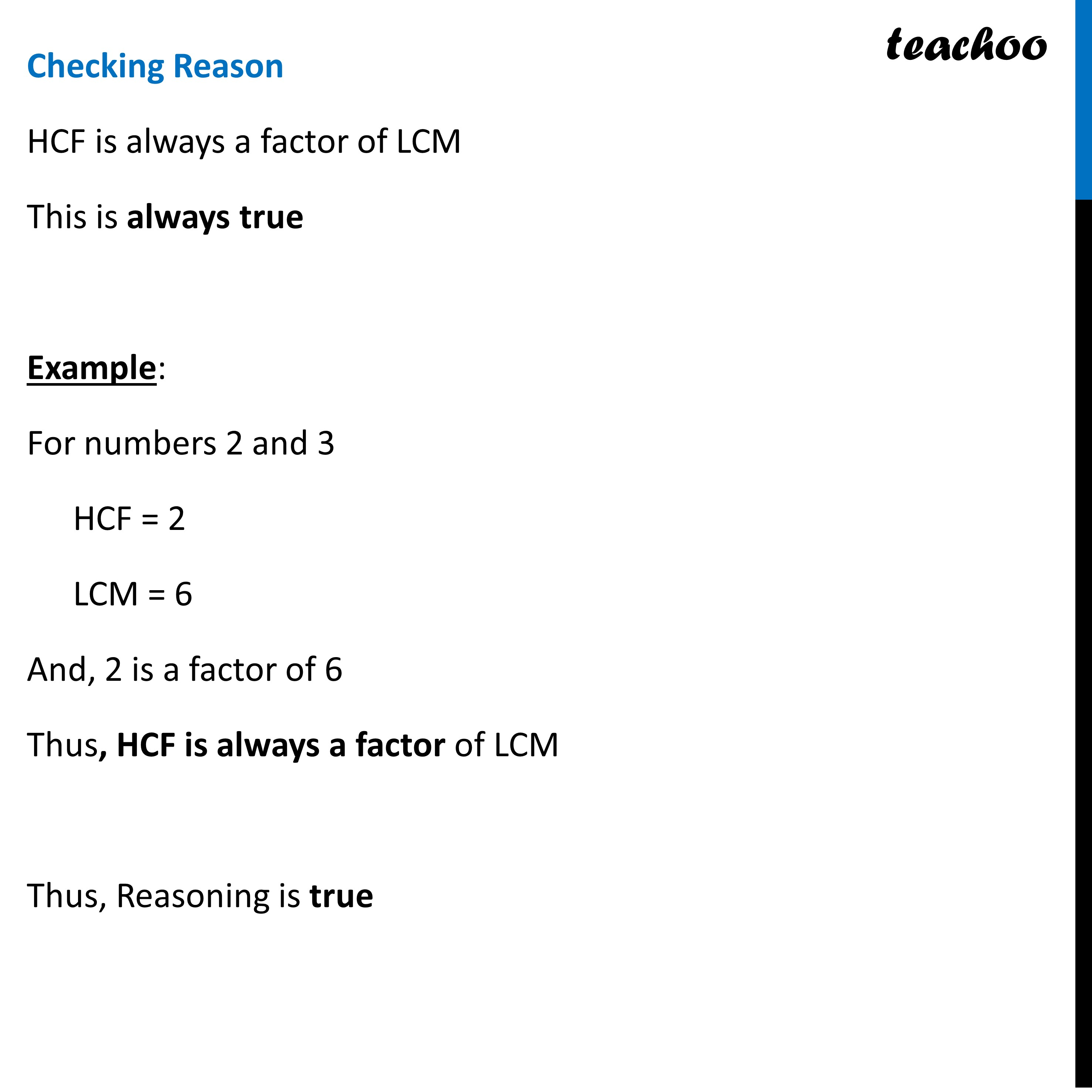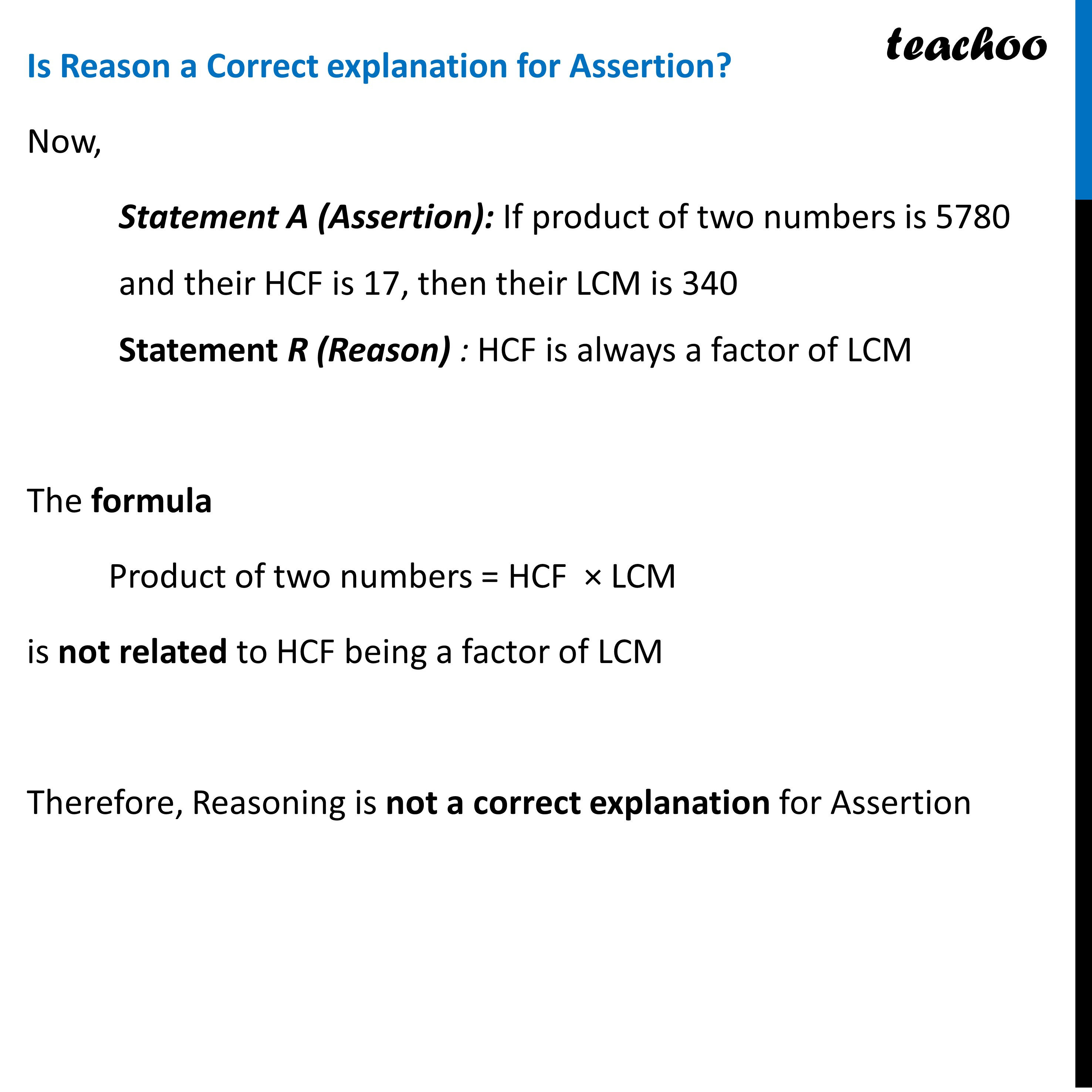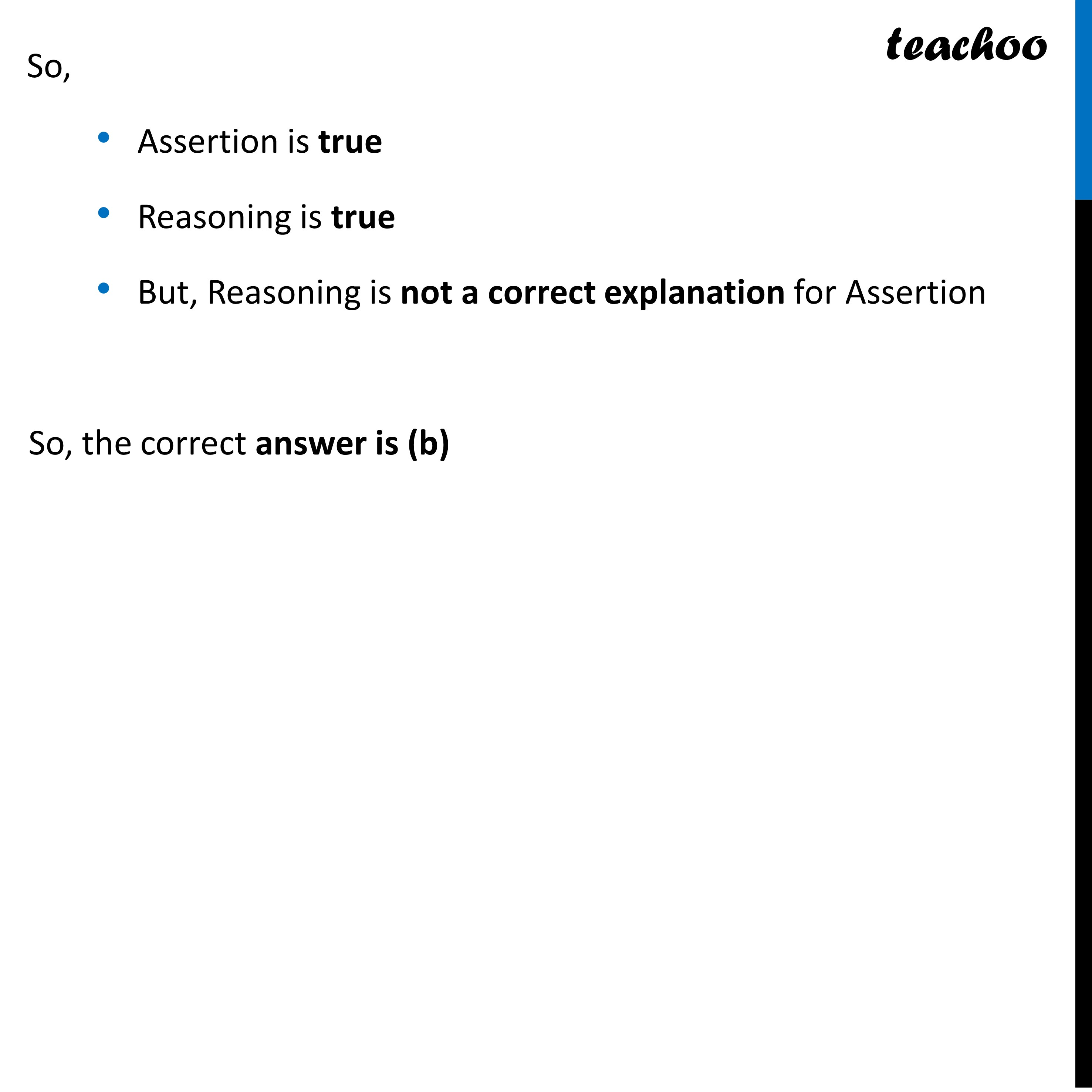Learn in your speed, with individual attention - Teachoo Maths 1-on-1 Class

### Transcript

Question 19 Statement A (Assertion): If product of two numbers is 5780 and their HCF is 17, then their LCM is 340 Statement R (Reason) : HCF is always a factor of LCM (a) Both assertion (A) and reason (R) are true and reason (R) is the correct explanation of assertion (A) (b) Both assertion (A) and reason (R) are true and reason (R) is not the correct explanation of assertion (A) (c) Assertion (A) is true but reason (R) is false. (d) Assertion (A) is false but reason (R) is true.Checking Assertion Given Product of two numbers = 5780 HCF = 17 We know that Product of two numbers = HCF × LCM 5780 = 17 × LCM 5780/17 = LCM LCM = 5780/17 LCM = 340 Thus, Assertion is true Checking Reason HCF is always a factor of LCM This is always true Example: For numbers 2 and 3 HCF = 2 LCM = 6 And, 2 is a factor of 6 Thus, HCF is always a factor of LCM Thus, Reasoning is true Is Reason a Correct explanation for Assertion? Now, Statement A (Assertion): If product of two numbers is 5780 and their HCF is 17, then their LCM is 340 Statement R (Reason) : HCF is always a factor of LCM The formula Product of two numbers = HCF × LCM is not related to HCF being a factor of LCM Therefore, Reasoning is not a correct explanation for Assertion So, Assertion is true Reasoning is true But, Reasoning is not a correct explanation for Assertion So, the correct answer is (b)